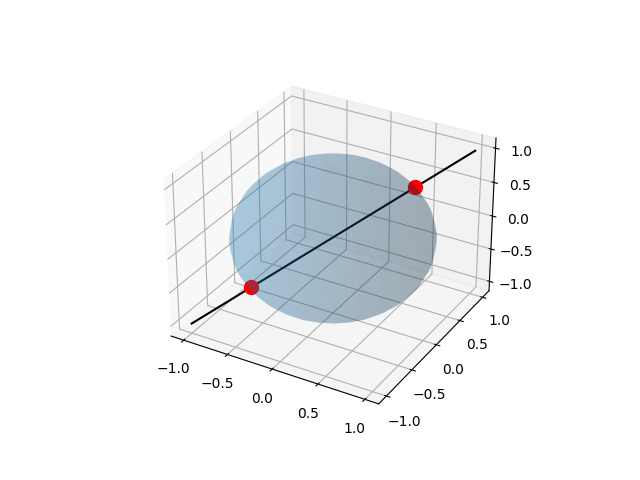# Sphere-Line Intersection¶Out:

```(<Figure size 640x480 with 1 Axes>, <Axes3DSubplot:>)
```

```from skspatial.objects import Line, Sphere
from skspatial.plotting import plot_3d

sphere = Sphere([0, 0, 0], 1)
line = Line([0, 0, 0], [1, 1, 1])

point_a, point_b = sphere.intersect_line(line)

plot_3d(
line.plotter(t_1=-1, c='k'),
sphere.plotter(alpha=0.2),
point_a.plotter(c='r', s=100),
point_b.plotter(c='r', s=100),
)
```

Total running time of the script: ( 0 minutes 0.206 seconds)

Gallery generated by Sphinx-Gallery